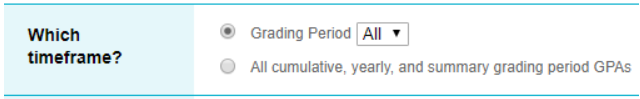# How do I calculate GPA?

In SchoolInsight, schools calculate student GPA based on report card grades.  This is typically done prior to finalizing report cards for distribution to parents.

Before calculating, you’ll need to make sure a few things are set up:

• Enable the option to calculate GPA
• Select a grade level range for cumulative GPA
• Check the box labeled “Used for Calculating GPA and Credits Earned”
• Make sure each letter grade has a corresponding GPA value
• (School District) Admin Main > Courses > Course Catalog
• Edit and mark each course as “Counts for GPA” as applicable
• Report card grades are entered for each course that counts towards GPA

Once you have those settings in order:

2. Click Calculate GPA
3. Select the time frame for calculation• Select the first option to calculate for underlying grading periods (Q1, Q2, T1, etc.) and also choose which grading period you’d like to calculate. These can be done individually or all at once.
• Select the second option to calculate for summary grading periods (Sem #1, Sem #2, Final), yearly and cumulative GPAs.
4. Select the grade level you’d like to calculate GPA for. Cumulative will calculate for the range defined earlier in (School District) Admin Main > Grade Reporting > Options
5. Click Calculate GPA

These calculations can take some time, and it is usually best practice to calculate all the individual grading period GPAs first, followed by the cumulative, yearly and summary grading period GPAs.